# The SORT Function in Excel

The SORT function is introduced in Excel 365 online. It is an dynamic array function that sort the given array into ascending and descending orders. This function is not available in Excel 2016 and older versions.
In this article, we will learn how to use the SORT function to sort arrays and tables in excel.
Syntax of SORT Function

=SORT(array,[sort_index],[sort_order],[by_col])

Array : It is the reference of array or range that you want to sort.

[sort_index] : The column number in the two dimensional array by which you want to sort the range. By Default it is 1.

[sort_order] : The order by which you want to sort the array. For ascending it is 1 and for descending it is -1. By default it is 1.

[by_col] : Set it True(1) if you want to sort an horizontal array. By default it False(0) for Vertical data.

Let’s understand this SORT function with some examples
Example 1: Sort Entire Table in Ascending Order By First Column.
Here I have this sample data table that I want to sort with the first column in ascending order.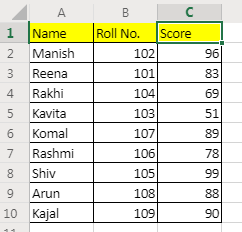The formula is simple:

=SORT(A2:C10)

It will return a dynamic array and will fill the sheet with the resulting sorted array.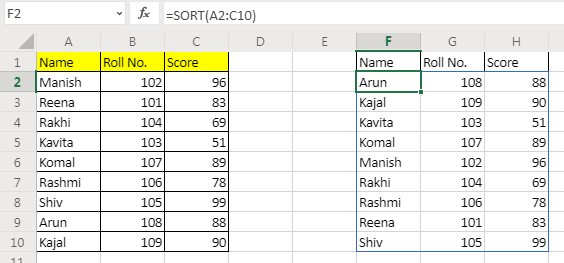Example 2: Sort Table By Column 2 in Descending Order
In our table, the second column is the Roll No. We need to sort this table by roll no. in reverse order.
The formula for sorting array in descending order of column 2 will be:

=SORT(A2:C10,2,-1)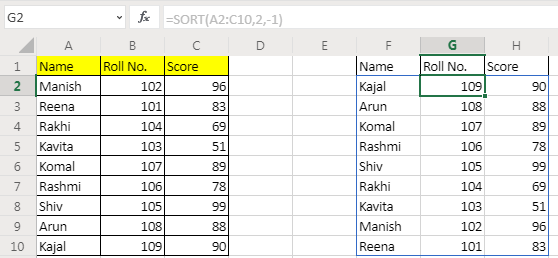Example 3: Sort the Horizontal Data in Ascending Order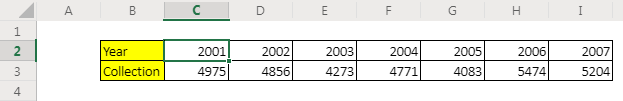Here, I have data of different years. I want to sort this data by collection in ascending order. The SORT formula for this will be:

=SORT(C2:I3,2,,1)

Here, we wanted to sort horizontally, so we set by_col variable to 1(TRUE). Since we wanted sort by the second row, the sort index is set to 2. We left the order_by to be the default.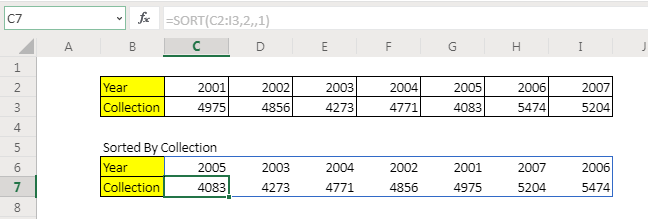How it works

The formula works automatically in the background to sort the given arrays.

Note: This function is only available in office 365 online version. It is not available in Excel 2016 or older versions. To sort arrays in Excel 2016 and older versions use these sorting formulas.

So yeah guys, this was a quick tutorial of how to use SORT function to sort arrays in Excel. I hope I was explanatory enough. If you have any doubts regarding this query or any other EXCEL VBA related query, ask them in the comments section below.

Related Articles:

Sort numbers using Excel SMALL Function | To sort numbers using formula in Excel 2016 and older, you can use the SMALL function with other helping functions.

Sort Numeric Values with Excel RANK Function | To sort the numeric values we can use the Rank function. The formula is

Excel Formula to Sort Text | To sort text values using formula in excel we simply use the COUNTIF function. Here is the formula

Popular Articles:

50 Excel Shortcuts to Increase Your Productivity | Get faster at your task. These 50 shortcuts will make you work even faster on Excel.

The VLOOKUP Function in Excel | This is one of the most used and popular functions of excel that is used to lookup value from different ranges and sheets.

COUNTIF in Excel 2016 | Count values with conditions using this amazing function. You don't need to filter your data to count specific values. Countif function is essential to prepare your dashboard.

How to Use SUMIF Function in Excel | This is another dashboard essential function. This helps you sum up values on specific conditions.

#### Related Excel Tips

Terms and Conditions of use

The applications/code on this site are distributed as is and without warranties or liability. In no event shall the owner of the copyrights, or the authors of the applications/code be liable for any loss of profit, any problems or any damage resulting from the use or evaluation of the applications/code.